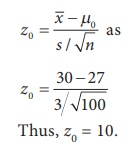Home | | Statistics 12th Std | Test of Hypotheses for Population Mean (Population Variance is Unknown)

# Test of Hypotheses for Population Mean (Population Variance is Unknown)

General Procedure for Test of Hypotheses for Population Mean (Population Variance is Unknown): Procedure Steps, Example Solved Problems

TEST OF HYPOTHESES FOR POPULATION MEAN (POPULATION VARIANCE IS UNKNOWN)

### Procedure:

Step 1 : Let µx and σx2 be respectively the mean and the variance of the population under study, where σ2 is unknown. If µ0 is an admissible value of µ, then frame the null hypothesis as H0: µ = µ0 and choose the suitable alternative hypothesis from

(i) H1: µ ≠ µ0 (ii) H1: µ > µ0 (iii) H1: µ < µ0

Step 2 : Let (X1, X2, …, Xn) be a random sample of n observations drawn from the population, where n is large (n ≥ 30).

Step 3 : Specify the level of significance, α.

Step 4 : Consider the test statistic Z =under H0, whereand S are the sample mean and sample standard deviation respectively. It may be noted that the above test statistic is obtained from Z considered in the test described in Section 1.9 by substituting S for σ.

The approximate sampling distribution of the test statistic under H0 is the N(0,1) distribution.

Step 5 : Calculate the value of Z for the given sample (x1, x2, ..., xn) as.  Here,and s are respectively the values ofand S calculated for the given sample.

Step 6: Find the critical value, ze, corresponding to α and H1 from the following tableStep 7: Decide on H0 choosing the suitable rejection rule from the following table corresponding to H1.### Example 1.9

A motor vehicle manufacturing company desires to introduce a new model motor vehicle. The company claims that the mean fuel consumption of its new model vehicle is lower than that of the existing model of the motor vehicle, which is 27 kms/litre. A sample of 100 vehicles of the new model vehicle is selected randomly and their fuel consumptions are observed. It is found that the mean fuel consumption of the 100 new model motor vehicles is 30 kms/litre with a standard deviation of 3 kms/litre. Test the claim of the company at 5% level of significance.

### Solution:

Step 1 : Let the fuel consumption of the new model motor vehicle be assumed to be distributed according to a distribution with mean and standard deviation respectively μ and σ. The null and alternative hypotheses are

Null hypothesis H0: μ = 27

i.e., the average fuel consumption of the company’s new model motor vehicle is not significantly different from that of the existing model.

Alternative hypothesis H1: μ > 27

i.e., the average fuel consumption of the company’s new model motor vehicle is significantly lower than that of the existing model. In other words, the number of kms by the new model motor vehicle is significantly more than that of the existing model motor vehicle.

Step 2 : Data:

The given sample information are

Size of the sample (n) = 100. Hence, it is a large sample.

Sample mean ()= 30

Sample standard deviation(s) = 3

Step 3 : Level of significance

α= 5%

Step 4 : Test statistic

The test statistic under H0 isSince n is large, the sampling distribution of Z under H0 is the N(0,1) distribution.

Step 5 : Calculation of Test Statistic

The value of Z for the given sample information is calculated fromStep 6 : Critical Value

Since H1 is a one-sided (right) alternative hypothesis, the critical value at α = 0.05 is ze = z0.05 = 1.645.

Step 7 : Decision

Since H1 is a one-sided (right) alternative, elements of the critical region are defined by the rejection rule z0 > ze = z 0.05. Thus, it is a right -tailed test. Since, for the given sample information, z0 = 10 > z e = 1.645, H0 is rejected.

Tags : Procedure Steps, Example Solved Problems | Statistics , 12th Statistics : Chapter 1 : Tests of Significance - Basic Concepts and Large Sample Tests
Study Material, Lecturing Notes, Assignment, Reference, Wiki description explanation, brief detail
12th Statistics : Chapter 1 : Tests of Significance - Basic Concepts and Large Sample Tests : Test of Hypotheses for Population Mean (Population Variance is Unknown) | Procedure Steps, Example Solved Problems | Statistics